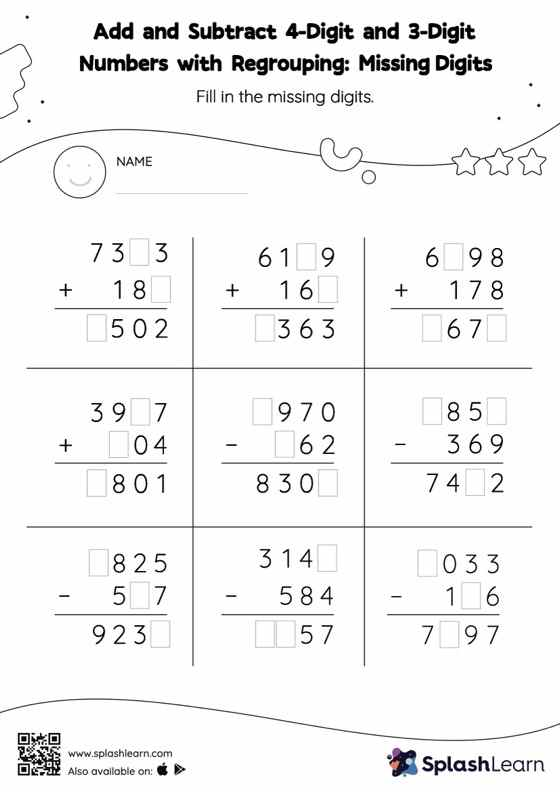# Add and Subtract 4-Digit and 3-Digit Numbers with Regrouping: Missing Digits Worksheet

Home > Add and Subtract 4-Digit and 3-Digit Numbers with Regrouping: Missing DigitsIn this worksheet, students must find the missing number using the relationship between addition and subtraction. To solve add and subtract 4-digit and 3-digit numbers with regrouping worksheet, they must also regroup the numbers. In each problem, the numbers are laid out in the vertical format. In this format, pair of digits in each successive place are tackled one by one. This helps students follow a structured approach.